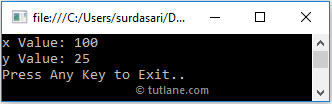# C# Out Parameter with Examples

In c#, out keyword is used to pass arguments to the method as a reference type. The `out` keyword same as the `ref` keyword, but the only difference is `out` doesn’t require a variable to be initialized before we pass it as an argument to the method. Still, the variable must be initialized in called method before it returns a value to the calling method.

The out parameter in c# is also useful to return more than one value from the methods in the c# programming language.

## Declaration of C# Out Parameter

Following is a simple example of using `out` parameters in c# programming language.

int x; // No need to initialize the variable
Multiplication(out x);

If you observe the above declaration, we just declared a variable x and passed it to the method using `out` parameter without assigning any value. Still, as discussed, the variable must be initialized in called method before it returns a value to the calling method.

To use `out` parameter in the c# application, both the method definition and the calling method must explicitly use the `out` keyword.

## C# Out Parameter Example

Following is the example of passing an `out` parameter to the method in the c# programming language.

using System;

namespace Tutlane
{
class Program
{
static void Main(string[] args)
{
int x;
Multiplication(out x);
Console.WriteLine("Variable Value: {0}", x);
Console.WriteLine("Press Enter Key to Exit..");
}
public static void Multiplication(out int a)
{
a = 10;
a *= a;
}
}
}

If you observe the above example, we declared a variable x and passed it to a Multiplication method by using `out` keyword without initializing the value. However, the called method (Multiplication) is initializing the value before returning the value to the calling method.

When we execute the above c# program, we will get the result below.If you observe the above result, the changes we did for a variable in the Multiplication method have also reflected the calling method.

## C# Multiple Out Parameters Example

Following is the example of using multiple `out` parameters in the c# programming language.

using System;

namespace Tutlane
{
class Program
{
static void Main(string[] args)
{
int x, y;
Multiplication(out x, out y);
Console.WriteLine("x Value: {0}", x);
Console.WriteLine("y Value: {0}", y);
Console.WriteLine("Press Enter Key to Exit..");
}
public static void Multiplication(out int a, out int b)
{
a = 10;
b = 5;
a *= a;
b *= b;
}
}
}

If you observe the above example, we defined two variables (x, y) and passed them to the Multiplication method using `out` parameters.

When we execute the above c# program, we will get the result below.If you observe the above result, the changes we did for variables in the Multiplication method have also been reflected in the calling method.

This is how we can use `out` parameter in c# programming language to pass arguments to the method as a reference type in c# programming language based on our requirements.